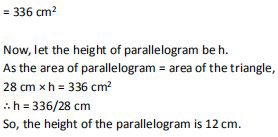# NCERT Solutions Class 9 Mathematics Solutions for Heron’s Formula - Exercise 12.2 in Chapter 12 - Heron’s Formula

A triangle and a parallelogram have the same base and the same area. If the sides of the

triangle are 26 cm, 28 cm and 30 cm, and the parallelogram stands on the base 28 cm, find

the height of the parallelogram

Given,

It is given that the parallelogram and triangle have equal areas.

The sides of the triangle are given as 26 cm, 28 cm and 30 cm.

So, the perimeter = 26 + 28 + 30 = 84 cm

And its semi perimeter = 84/2 cm = 42 cm

Now, by using Heron's formula, the area of the triangle =

√[s (s-a) (s-b) (s-c)]

\begin{array}{l} =v[42(42-26)(46-28)(46-30)] \mathrm{cm}^{2} \\ =\sqrt{[46 \times 16 \times 14 \times 16] \mathrm{cm}^{2}} \end{array}Video transcript
"hello students welcome to leader q a video session i am sef your math tutor and question for today is a triangle and a parallelogram have the same base and same area if the sides of the triangle are 26 centimeter 28 centimeter and 30 centimeter and the parallelogram stands on the base of 28 centimeter find the height of the parallelogram now it is given that the parallelogram and the triangle have equal areas the sides of the triangle are given as 26 centimeter and 28 centimeter and 30 centimeter so the perimeter will be equal to 26 plus 28 plus 30. we have indicated parameter as p and hence that is 84 centimeter the semi perimeter can be found now to find semi perimeter we have to add all the side and divide it by two so semi perimeter of triangle as that is equal to 26 plus 28 plus 30 upon 2 so s will be equal to 42 centimeter now you can find the area to find the area we have to use the harold's formula so by using heron's formula the area of the triangle can be given by let it indicate as a root of s s minus a s minus b and s minus c a b c are the sides so as is you have found that is 42 centimeter so 42 42 minus 26 42 minus 28 and 42 minus 30 that will be equal to root of 46 into 16 into 14 into 16 and that will be centimeter square because it is area and that will give you area will be equal to 336 centimeter square now let the height of parallelogram be h as the area of parallelogram is given will be equal to area of triangle we can compare both and find height so we will continue over here it is given that area of parallelogram will be equal to area of triangle and hence 28 into h that is base into height that is the area of parallelogram is equal to area we have found to be of triangle that is 336 so height will be equal to 336 divided by 28. height of the parallelogram will be 12 centimeter and that is our final answer so if you have any query you can drop it in our comment section and subscribe to lido for more such q and a thank you for watching "
Related Questions

Lido

Courses

Teachers

Book a Demo with us

Syllabus

Maths
CBSE
Maths
ICSE
Science
CBSE

Science
ICSE
English
CBSE
English
ICSE
Coding

Terms & Policies

Selina Question Bank

Maths
Physics
Biology

Allied Question Bank

Chemistry
Connect with us on social media!